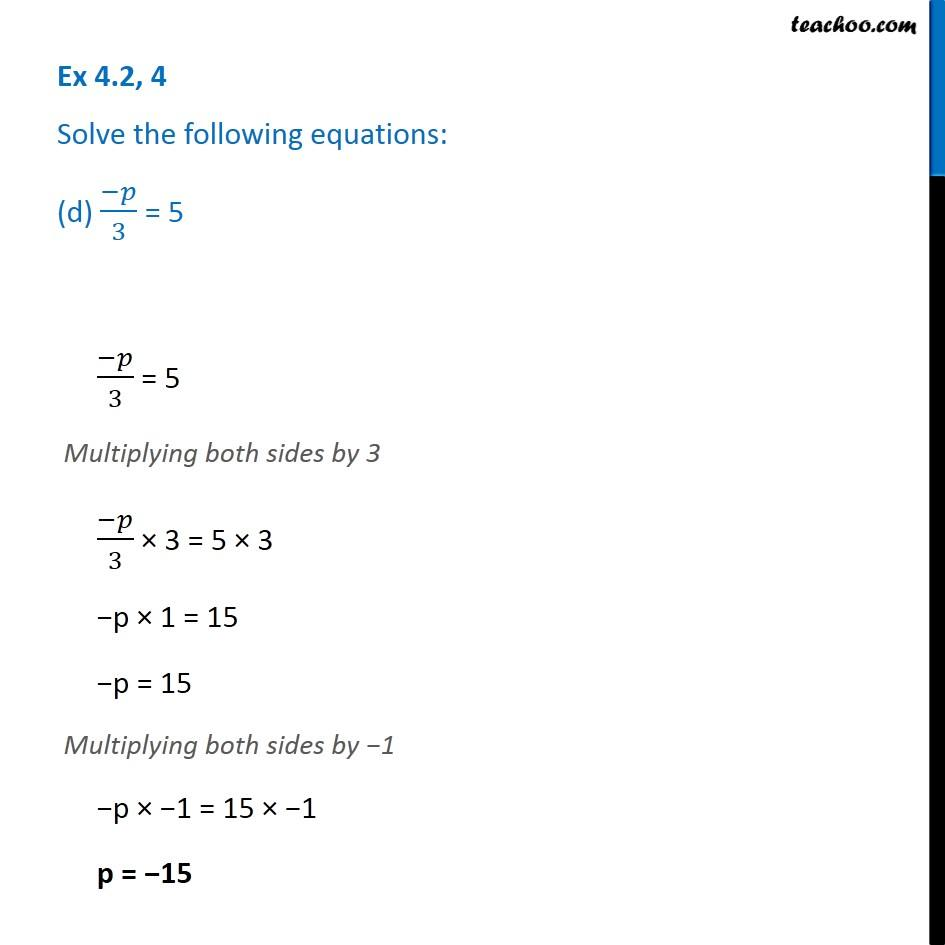Ex 4.2

Chapter 4 Class 7 Simple Equations
Serial order wiseLearn in your speed, with individual attention - Teachoo Maths 1-on-1 Class

### Transcript

Ex 4.2, 4 Solve the following equations: (d) (−𝑝)/3 = 5(−𝑝)/3 = 5 Multiplying both sides by 3 (−𝑝)/3 × 3 = 5 × 3 −p × 1 = 15 −p = 15 Multiplying both sides by −1 −p × −1 = 15 × −1 p = −15